# The F_un folklore

Posted by on Sep 22, 2008 in graduate, KapranovSmirnov, Manin19951 comment

This text was posted on June, 7th 2008 at neverendingbooks

All esoteric subjects have their own secret (sacred) texts. If you opened the Da Vinci Code (or even better, the original The Holy blood and the Holy grail) you will known about a mysterious collection of documents, known as the “Dossiers secrets“, deposited in the BibliothÃ¨que nationale de France on 27 April 1967, which is rumoured to contain the mysteries of the Priory of Sion, a secret society founded in the middle ages and still active today…

The followers of F-un, forthe field of one element, have their own collection of semi-secret texts, surrounded by whispers, of which they try to decode every single line in search of enlightenment. Fortunately, you do not have to search the shelves of the Bibliotheque National in Paris, but the depths of the internet to find them as huge, bandwidth-unfriendly, scanned documents.The first are the lecture notes “Lectures on zeta functions and motives” by Yuri I. Manin of a course given in 1991.

One can download a scanned version of the paper from the homepage of Katia Consani as a huge 23.1 Mb file. Of F-un relevance is the first section “Absolute Motives?” in which

“…we describe a highly speculative picture of analogies between arithmetics overand over, cast in the language reminiscent of Grothendieck’s motives. We postulate the existence of a category with tensor product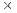whose objects correspond not only to the divisors of the Hasse-Weil zeta functions of schemes over, but also to Kurokawa’s tensor divisors. This neatly leads to teh introduction of an “absolute Tate motive”, whose zeta function is, and whose zeroth power is “the absolute point” which is teh base for Kurokawa’s direct products. We add some speculations about the role ofin the “algebraic geometry over a one-element field”, and in clarifying the structure of the gamma factors at infinity.” (loc.cit. p 1-2)

I’d welcome links to material explaining this section to people knowing no motives.The second one is the unpublished paper “Cohomology determinants and reciprocity laws : number field case” by Mikhail Kapranov and A. Smirnov.

This paper features in blog-posts at the Arcadian Functor, in John Baez’ Weekly Finds and in yesterday’s post at Noncommutative Geometry.

You can download every single page (of 15) as a separate file from here. But, in order to help spreading the Fun-gospel, I’ve made these scans into a single PDF-file which you can download as a 2.6 Mb PDF. In the introduction they say :

“First of all, it is an old idea to interpret combinatorics of finite sets as thelimit of linear algebra over the finite field. This had lead to frequent consideration of the folklore object, the “field with one element”, whose vector spaces are just sets. One can postulate, of course, that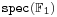is the absolute point, but the real problem is to develop non-trivial consequences of this point of view.”

They manage to deduce higher reciprocity laws in class field theory within the theory ofand its field extensions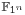. But first, let us explain how they define linear algebra over these absolute fields.

Here is a first principle : in doing linear algebra over these fields, there is no additive structure but only scalar multiplication by field elements. So, what are vector spaces over the field with one element? Well, as scalar multiplication with 1 is just the identity map, we have that a vector space is just a set. Linear maps are just set-maps and in particular, a linear isomorphism of a vector space onto itself is a permutation of the set. That is, linear algebra overis the same as combinatorics of (finite) sets.

A vector space overis just a set; the dimension of such a vector space is the cardinality of the set. The general linear groupis the symmetric group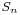, the identification via permutation matrices (having exactly one 1 in every row and column)

Some people prefer to view anvector space as a pointed set, the special element being the ‘origin’but asdoesnt have a zero, there is also no zero-vector. Still, in later applications (such as defining exact sequences and quotient spaces) it is helpful to have an origin. So, let us denote for any setby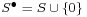. Clearly, linear maps between such ‘extended’ spaces must be maps of pointed sets, that is, sending.

The field with one elementhas a field extension of degree n for any natural number n which we denote byand using the above notation we will define this field as :withthe group of all n-th roots of unity. Note that if we choose a primitive n-th root, thenis the cyclic group of order n.

Now what is a vector space over? Recall that we only demand units of the field to act by scalar multiplication, so each ‘vector’determines an n-set of linear dependent vectors. In other words, any-vector space is of the formwitha set of which the groupacts freely. Hence,haselements and there are exactly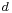orbits for the action ofby scalar multiplication. We callthe dimension of the vectorspace and a basis consists in choosing one representant for every orbits. That is,is a basis if (and only if).

So, vectorspaces are free-sets and hence linear mapsis a-map. In particular, a linear isomorphism of, that is an element ofis abijection sending any basis elementfor a permutation.

An-vectorspaceis a free-setofelements. The dimensionand the general linear groupis the wreath product ofwith, the identification as matrices with exactly one non-zero entry (being an n-th root of unity) in every row and every column.

This may appear as a rather sterile theory, so let us give an extremely important example, which will lead us to our second principle for developing absolute linear algebra.

Letbe a prime power and letbe the finite field withelements. Assume that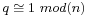. It is well known that the group of units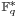is cyclic of orderso by the assumption we can identifywith a subgroup of.

Then,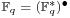is an-vectorspace of dimension. In other words,is an-algebra. But then, any ordinary-vectorspace of dimension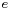becomes (via restriction of scalars) an-vector space of dimension.

Next time we will introduce more linear algebra definitions (including determinants, exact sequences, direct sums and tensor products) in the realm the absolute fieldsand remarkt that we have to alter the known definitions as we can only use the scalar-multiplication. To guide us, we have the second principle : all traditional results of linear algebra overmust be recovered from the new definitions under the vector-space identificationwhen. (to be continued)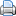Print This Post